# KVPY-SA 2018 Chemistry Paper With Solutions

KVPY-SA 2018 Paper with Solutions Chemistry is a perfect resource which helps the candidates in understanding different methods of solving a particular problem. Candidates can download the previous years’ question papers of KVPY to figure out the frequently occurring questions in the main exams. Practising the KVPY previous year papers will guide the students to attempt each question with the best possible approach and arrive at the solution as soon as possible to save time. Solving these papers with solutions will help the students understand the concepts and coverage for the upcoming exam.

### KVPY SA 2018 - Chemistry

Question 1: The number of water molecules in 250 mL of water is closest to

[Given: Density of water is 1.0 g mL–1; Avogadro’s number = 6.023 × 1023]

1. a. 83.6 × 1023
2. b. 13.9 × 1023
3. c. 1.5 × 1023
4. d. 33.6 × 1023

Solution:

Given Density of water = 1 g / ml

The volume of water = 250 ml

We know that d = m / v

m = d × v

Mass of H2O = 250 × 1 = 250 g

Mw of H2O = 18 g mol–1

Moles of H2O = wt / Mw = 250 / 18 = 13.89 moles

We know that in 1 mole, the number of H2O

Molecules = NA = 6.023 × 1023 molecules

In 13.89 moles, the no of H2O Molecules

= 6.023 × 1023 × 13.89

= 83.6 × 1023 Molecules

Therefore, the correct option is (a).

Question 2: Among the following, the correct statement is

1. a. pH decreases when solid ammonium chloride is added to a dilute aqueous solution of NH3
2. b. pH decreases when solid sodium acetate is added to a dilute aqueous solution of acetic acid
3. c. pH decreases when solid NaCl added to a dilute aqueous solution of NaOH
4. d. pH decreases when solid sodium oxalate is added to a dilute aqueous solution of oxalic acid

Solution:

dil. aq. Soln of NH3 → NH3 + H2O ⇒ NH4OH (Weak Base)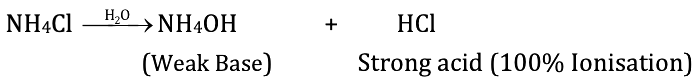If we add NH4Cl, It is an acidic salt because it is prepared by strong acid (HCl) weak base (NH4OH)

So overall pH ↓ because the concentration of H+ ion ↑

pH ∝ (1 / [H+]) or [H+] ↑ pH ↓

Therefore, the correct option is (a).

Question 3: The solubility of BaSO4 in pure water (in g L–1) is closest to [Given: Ksp for BaSO4 is 1.0 × 10–10 at 25°C. The molecular weight of BaSO4 is 233 g mol–1]

1. a. 1.0 × 10–5
2. b. 1.0 × 10–3
3. c. 2.3 × 10–5
4. d. 2.3 × 10–3

Solution:

Given, Ksp of BaSO4 = 1.0 × 10–10

Molecular weight of BaSO4 = 233 g mol–1 {Moles (n) = weight (g) / molecular weight}

BaSo4 ⇌ Ba+2 + SO42-

Ksp (BaSO4) = S2

S = √Ksp = √1 * 10-10 = 10-5 mol L-1

S = 10–5 × Mw = 10–5 × 233 g L–1

Moles (n) = wt(g) / mw

Wt (g) = n × Mw

S = 2.33 × 10–3 gL–1

Therefore, the correct option is (d).

Question 4: Among the following, the INCORRECT statement is

1. a. No two electrons in an atom can have the same set of four quantum numbers
2. b. The maximum number of electrons in the shell with a principal quantum number, n, is equal to n2+2
3. c. Electrons in an orbital must have opposite spin
4. d. In the ground state, atomic orbitals are filled in the order of their increasing energies

Solution:

According to Pauli’s Exclusion Principle, no two e–s in the same atom can have identical values for all four of their quantum numbers.

Ex for He → 1s2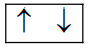1ste → n = 1, l = 0, m = 0 s = + 1 / 2

IInde → n = 1, l = 0, m = 0 s = – 1 / 2

(B) The Maximum number of electrons in the shell with principal quantum number ‘n’ is equal to 2n2

(C) Electron in an orbital must have opposite

Spin Example(D) In ground state, atomic orbitals are filled in the order of their ↑ energy [see (n + l ) Rule)

1s > 2s > 2p > 3s……

Therefore, the correct option is (b).

Question 5: A container of volume 2.24 L can withstand a maximum pressure of 2 atm at 298 K before exploding. The maximum amount of nitrogen (in g) that can be safely put in this container at this temperature is closest to

1. a. 2.8
2. b. 5.6
3. c. 1.4
4. d. 4.2

Solution:

Given

v = 2.24 L

T = 298 K

p = 2 atm

R = 0.0821 atm mol–1 k–1

from ideal gas equation Pv = nRT,

Moles of N2 = Pv / RT = [2 * 2.24] / [0.0821 * 298] = 0.1831 moles.

The molecular weight of N2 = 28 g mol–1

Moles = Weight / Molecular weight

Weight (g) = moles × Molecular Weight (g mol–1)

Wt of N2 = 0.1831 × 28 ≅ 5.6 g

Therefore, the correct option is (B).

Question 6: The compound is shown below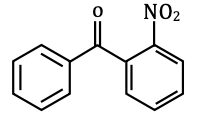Can be readily prepared by Friedel-Crafts reaction between

1. a. benzene and 2-nitrobenzoyl chloride
2. b. benzyl chloride and nitrobenzene
3. c. nitrobenzene and benzoyl chloride
4. d. benzene and 2-nitrobenzyl chloride

Solution:

The preparation of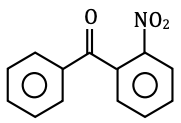via Friedel craft Reaction follows as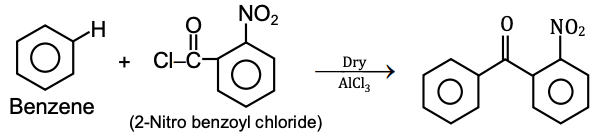Therefore, the correct option is (a).

Question 7: The correct statement about the following compounds is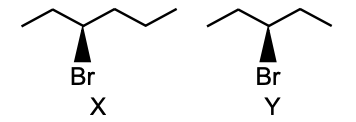1. a. both are chiral
2. b. both are achiral
3. c. X is chiral and Y is achiral
4. d. X is achiral and Y is chiral

Solution:

Asymmetric centre → sp3 carbon with 4 different groups attached.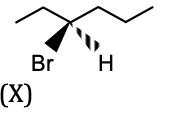Here all 4 groups are different.

Hence it is a chiral carbon.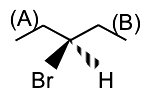Here two groups are the same.

→ Hence it is not a chiral compound.

So, here X is chiral & Y is achiral compound.

Therefore, the correct option is (c).

Question 8: The most acidic proton and the strongest nucleophilic nitrogen in the following compound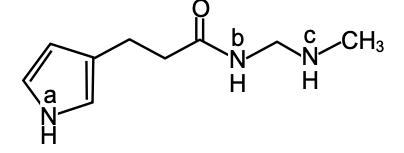Respectively, are

1. a. Na–H; Nb
2. b. Nb–H; Nc
3. c. Na -H; Nc
4. d. Nc -H; Na

Solution: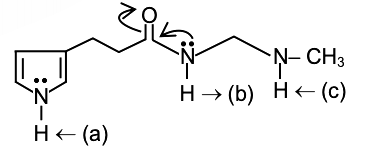Due to the resonance of N & O in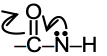. It is the most acidic.

In the case of (C) the lone pair of N is not involved in delocalization, hence it is the strongest Nucleophilic.

Therefore, the correct option is (b).

Question 9: The chlorine atom of the following compound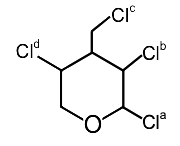That reacts most readily with AgNO3 to give a precipitate is

1. a. Cla
2. b. Clb
3. c. Clc
4. d. Cld

Solution: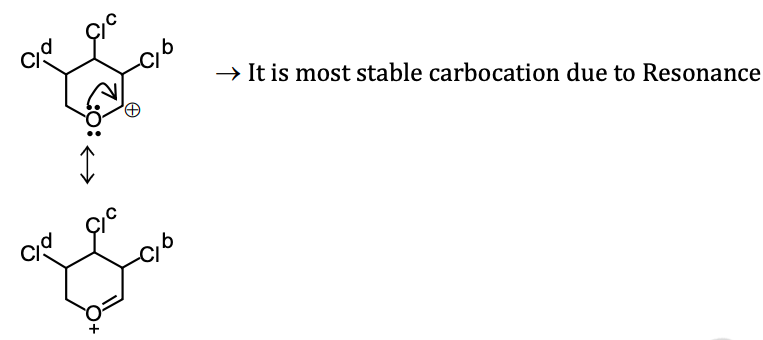Hence, it reacts most readily with AgNO3 to give a precipitate.

Therefore, the correct option is (a).

Question 10: Among the following sets, the most stable ionic species are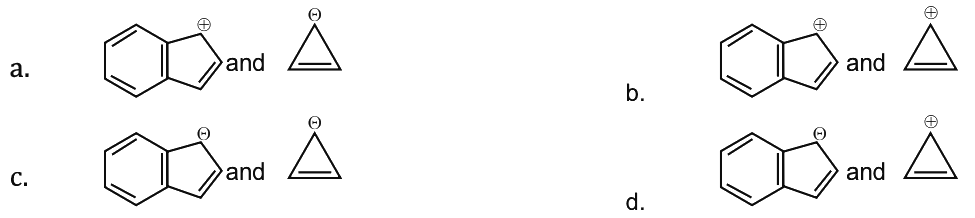Solution: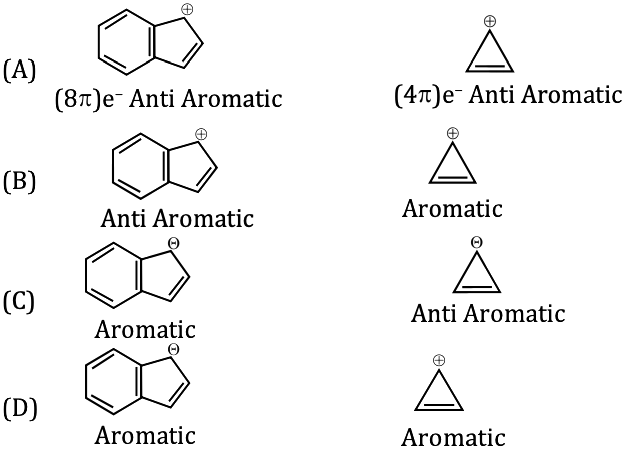Order of stability

Aromatic > Non Aromatic > Anti Aromatic

Hence option (D) correct because in the case of (D) both are aromatic.

Question 11: The correct order of energy of 2s orbitals in H, Li, Na and K, is

1. a. K < Na < Li < H
2. b. Na < Li < K < H
3. c. Na < K < H < Li
4. d. H < Na < Li < K

Solution:

As we go down the group IA, there is ↑ in the shell, so the size of atom ↑ & energy of 2s orbital ↓.

Hence the correct order is K < Na < Li < H.

Therefore, the correct option is (a).

Question 12: The hybridization of the xenon atom in XeF4 is

1. a. sp3
2. b. dsp3
3. c. sp3d2
4. d. d2sp3

Solution: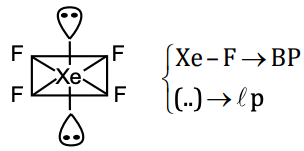In XeF4: → Xe has 8e its outermost shell (initial)

→ After the formation of XeF4

It has 4 bond pairs and 2 lone pairs as shown in the figure.

Hence steric number = lp + BP = 6

Thus the hybridization is SP3d2.

Therefore, the correct option is (c).

Question 13: The formal oxidation numbers of Cr and Cl in the ions Cr2O72– and ClO3– respectively, are

1. a. +6 and + 7
2. b. +7 and +5
3. c. +6 and + 5
4. d. +8 and + 7

Solution:

Filter paper soaked with KI turns brown when exposed to HNO3 vapour to libration of I2, the reaction follow as

6KI + 8HNO3 → 6KNO3 + 4H2O + 2NO + 3I2

Therefore, the correct option is (c).

Question 14: A filter paper soaked in salt X turns brown when exposed to HNO3 vapour. The salt X is –

1. a. KCl
2. b. KBr
3. c. KI
4. d. K2SO4

Solution:

Let the oxidation number of Cr = x

Cr2O72– ⇒ 2x + (–2) × 7 = –2

2x = 12

x = +6

Let the oxidation number of Cl = x

ClO3– → x +(–2) × 3 = –1

x = + 5

Therefore, the correct option is (c).

Question 15: The role of haemoglobin is to

1. a. store oxygen in muscles
2. b. transport oxygen to different parts of the body
3. c. convert CO to CO2
4. d. convert CO2 into carbonic acid

Solution:

The role of haemoglobin is to transport oxygen to different parts of the body. Therefore, the correct option is (b).

Question 16: Among the following, the species with identical bond order are

1. a. CO and O22–
2. b. O22– and CO
3. c. O22– and B2
4. d. CO and N2+

Solution:

According to MOT, bond order of all species are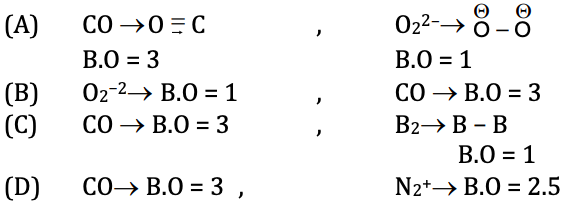Hence here identical bond order observed in the case of (C)

Bond order, O22– = B2 =1

Therefore, the correct option is (c).

Question 17: The quantity of heat (in J) required to raise the temperature of 1.0 kg of ethanol from 293.45 K to the boiling point and then change the liquid to vapour at that temperature is closest to [Given: Boiling point of ethanol 351.45 K Specific heat capacity of liquid ethanol 2.44 J g–1 K–1 Latent heat of vaporization of ethanol 855 J g–1]

1. a. 1.42 × 102
2. b. 9.97 × 102
3. c. 1.42 × 105
4. d. 9.97 × 105

Solution: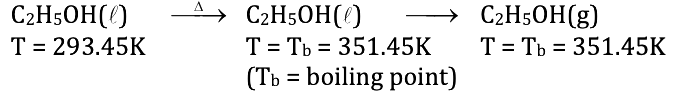Heat required (Q) = MsΔT + Heat of vaporization

= 103 × 2.44 [351.45 – 293.45] + 103(855)

= 103 [(2.44 × 58) +855]

= 103 [996.52]

so, Q= 9.97 × 105 J

Therefore, the correct option is (d).

Question 18: A solution of 20.2 of 1, 2-dibromopropane in MeOH upon heating with excess Zn produces 3.58 g of an unsaturated compound X. The yield (%) of X is closest to [Atomic weight of Br is 80]

1. a. 18
2. b. 85
3. c. 89
4. d. 30

Solution:

The reaction of Zn with 1, 2 dibromo propane in MeOH follow as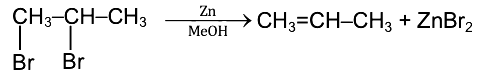Here 1 mol. Reactant give one mole product

→ M. wt of Reactant (1, 2 dibromo propane) = 202 g mol–1

→ wt of Reactant = 20.2 g

→ Mw of product = 42 g mol–1

wt of product = 3.58 g

We know moles = wt of substance / Mw of substance

Obtain moles of product = 3.58 / 42 = 0.085 moles

Moles of Reactant = 20.2 / 202 = 0.1 moles

→ Now according to equation theoretically,

Mole of Product = moles of Reactant = 0.1 moles

% yield = [(obtain Moles)/(theoretical moles)] * 100

= [0.085 / 0.1] * 100

= 85%

Therefore, the correct option is (b).

Question 19: The lower stability of ethyl anion compared to methyl anion and the higher stability of ethyl radical compared to the methyl radical, respectively, are due to

1. a. +I effect of the methyl group in ethyl anion and σ → p- orbital conjugation in ethyl radical
2. b. –I effect of the methyl group in ethyl anion and σ → σ conjugation in ethyl radical
3. c. +I effect of the methyl group in both cases
4. d. +I effect of the methyl group in ethyl anion and σ → σ conjugation in ethyl radical

Solution:

(1)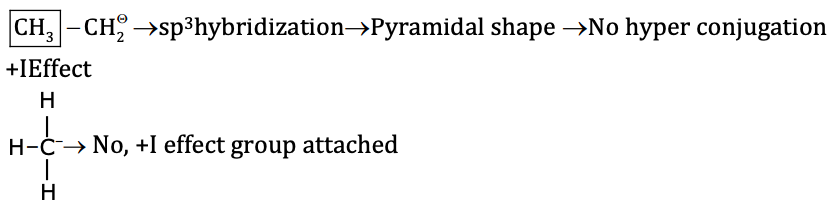In ethyl anion, methyl groups have + I effect which ↑ the e density on carbanion and decrease the stability.

(2)Due to σ – p orbital conjugation (Hyperconjugation), it is more stable compared to CH3 radical.

A number of α H ↑ stability of Radical ↑. Because of the number of hyperconjugation structure ↑ and energy of the molecule ↓.

Therefore, the correct option is (a).

Question 20: The F – Br-F bond angles in BrF5 and the Cl – P – Cl bond angles in PCl5, respectively, are

1. a. identical in BrF5 but non-identical in PCl5
2. b. identical in BrF5 and identical in PCl5
3. c. non-identical in BrF5 but identical in PCl5
4. d. non-identical in BrF5 and non-identical in PCl5

Solution:

(1) BrF5 → [7 + 5] / 2 = 6 → sp3d2 Hybridisation

n → lp + Bp

6 = 5 + lp

lp = 1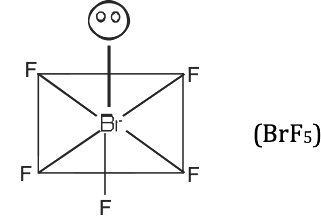Hence the shape of BrF5 is square pyramidal.

Hence here [F– Br – F] bond angle is non-identicals.

(2) PCl5 → [5 + 5] / 2 = 5→ Sp3d

[lp = n – BP = 5 – 5 = 0]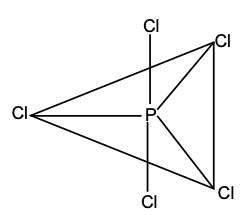Hence the shape is Trigonal bipyramidal.

→ (Cl – P – Cl) bond angle 120° & 90°.

So, here (Cl–P–Cl) bond angle is not identical.

Therefore, the correct option is (d).

### KVPY-SA 2018 Chemistry Paper with Solutions